### CEILING Function – Round to Multiple in Excel, VBA, G Sheets

This tutorial demonstrates how to use the Excel CEILING Function in Excel to round a number up.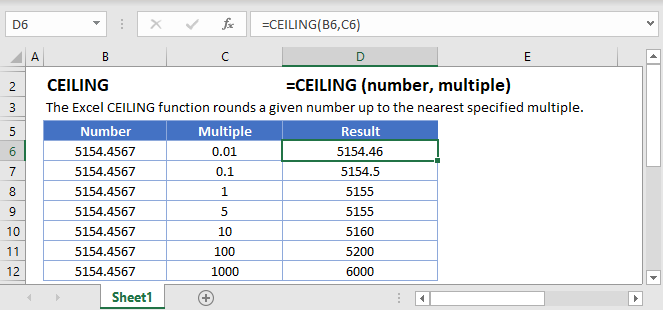## CEILING Function Overview

The CEILING Function Rounds a number up, to the nearest specified multiple.

To use the CEILING Excel Worksheet Function, select a cell and type: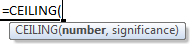(Notice how the formula inputs appear)

### CEILING Function Syntax and Inputs:

`=CEILING(number,significance)`

number – A number.

significance – The multiple to which to round the number. The sign much match the number. So if the number is negative, the significance must also be negative.

AutoMacro - VBA Code Generator

## How to use the CEILING Function

### Round up to Nearest 5, 50, or .5

To round up to the nearest 5, set the multiple argument to 5.

`=CEILING(B3,5)`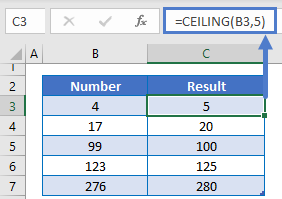Alternatively you can round up to the nearest .5 or 50 by changing the multiple argument.

```=CEILING(A2,0.5)
=CEILING(A2,5)
=CEILING(A2,50)```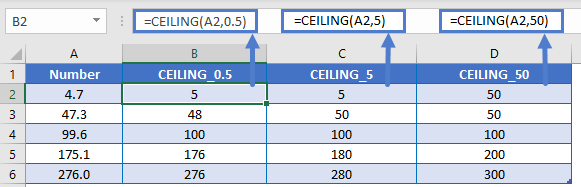### Round up to Nearest Quarter

You can also round a price up to the nearest quarter by setting multiple = .25.

`=CEILING(A2,0.25)`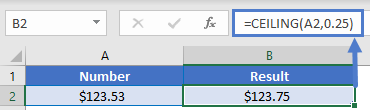### Round Time

The CEILING Function makes it easy to round up time. To round time up to a certain increment, just enter your desired unit of time using quotations. Here we will round up to the nearest 15 minutes.

`=CEILING(B3,"0:15")`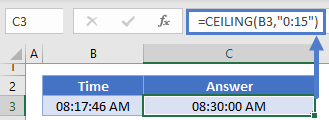## Other ROUND Functions / Formulas

Excel / Google Sheets contains many other round functions. Here are quick examples of each: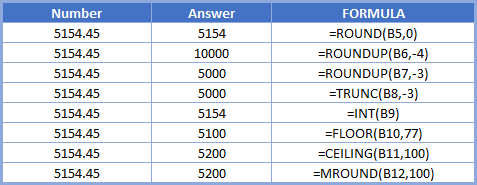Read our tutorials to learn more:

Below, we will point out a few functions in particular.

### FLOOR and MROUND

The FLOOR and MROUND Functions work exactly the same as the CEILING Function, except the FLOOR Function always rounds down and the MROUND Function always rounds to the nearest multiple.

```=MROUND(B3,5)
=FLOOR(B3,5)
=CEILING(B3,5)
```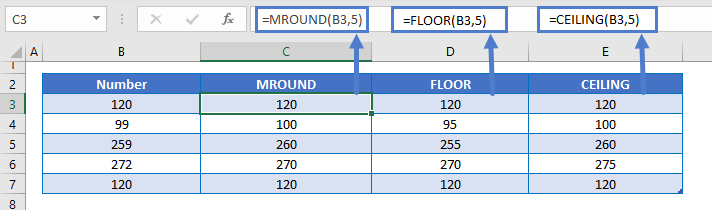### ROUNDUP Function

Instead of using the CEILING Function to round up to a multiple, you can use the ROUNDUP Function to round a number up to a certain number of digits.

`=ROUNDUP(B3,2)`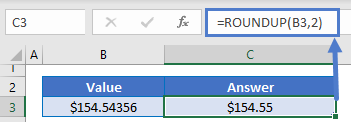## CEILING in Google Sheets

The CEILING Function works exactly the same in Google Sheets as in Excel: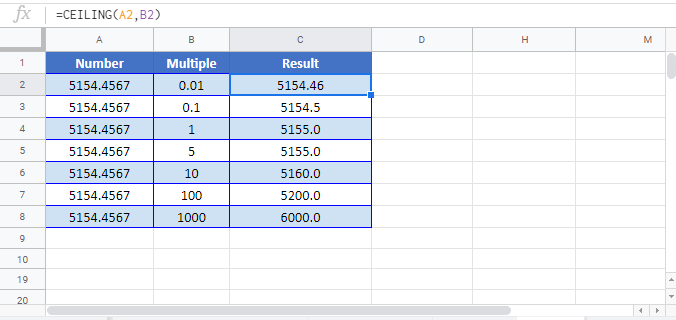## CEILING Examples in VBA

You can also use the CEILING function in VBA. Type:
`application.worksheetfunction.ceiling(number,significance)`

Executing the following VBA statements

```Range("C2") = Application.WorksheetFunction.Ceiling(Range("A2"), Range("B2"))
Range("C3") = Application.WorksheetFunction.Ceiling(Range("A3"), Range("B3"))
Range("C4") = Application.WorksheetFunction.Ceiling(Range("A4"), Range("B4"))
Range("C5") = Application.WorksheetFunction.Ceiling(Range("A5"), Range("B5"))
Range("C6") = Application.WorksheetFunction.Ceiling(Range("A6"), Range("B6"))
Range("C7") = Application.WorksheetFunction.Ceiling(Range("A7"), Range("B7"))
Range("C8") = Application.WorksheetFunction.Ceiling(Range("A8"), Range("B8"))
Range("C9") = Application.WorksheetFunction.Ceiling(Range("A9"), Range("B9"))```

will produce the following results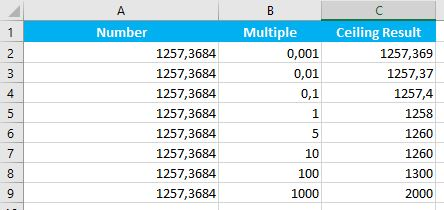For the function arguments (number, etc.), you can either enter them directly into the function, or define variables to use instead.

Return to the List of all Functions in Excel

## Excel Practice Worksheet

Practice Excel functions and formulas with our 100% free practice worksheets!

• Automatically Graded Exercises
• Learn Excel, Inside Excel!